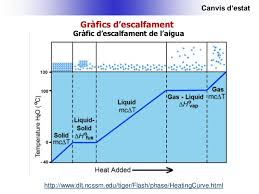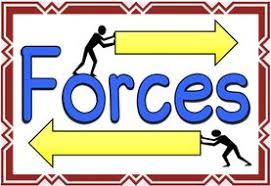Se muestran los artículos pertenecientes a Diciembre de 2018.

## 3ESO FQ 2018-2019 : Article 4 : CANVIS D'ESTAT.

Publicado: 05/12/2018 10:35 por easyfisquim en 3ESO FQ 2018-2019CANVIS DESTAT.

- Canvis destat dagregació de la matèria que anem a estudiar.

- Què és un canvi destat?.

- Experiment que hem fet al laboratori del canvi destat de VAPORITZACIÓ daigua
pura i daigua amb sal. Mesurem com augmenta la temperatura a lescalfar i com
aquesta roman constant durant el canvi destat de líquid a gas. Després el proper
dia construim la gràfica descalfament amb les dades obtenides per cada grup al
mesurar les temperatures a cada minut. Fixeu-vos a les fotos com (segons la qua-
litat del termòmetre emprat) laigua pura bull entorn als 100ºC mentres que laigua
amb sal el termòmetre marca fins a 105ºC com a temperatura de canvi destat al
principi, ja que després va canviat conforme es vaporitza laigua i es concentra més
la sal, ja que no és una substància pura sinó una mescla.

Foto de la gràfica descalfament construïda a partir de les dades
de temperatures obtingudes al laboratori.

- Pràctica de la sublimació del iode i de la sublimació inversa o regressiva fent servir
un matraç amb gel :

+  Resum o informe de la pràctica a la pissarra :   FOTO

- Pissarra amb els principals tipus de vaporitzacions : Evaporació i ebullició :

FOTO Pissarra :   Diferències entre evaporació i ebullició

- Canvi de la temperatura debullició d'un líquid pur amb laltura i la pressió :
( Cal veure el video i després escriure l'explicació que es dóna del perquè de l'experiment i procés ).

- Foto de l'esquema de la muntanya on la temperatura debullició d'un líquid pur
canvia amb l'altura i per tant també amb la pressió:

## 4ESO FQ English 2018-2019. Unit 2 FORCES. Article 4.

Publicado: 10/12/2018 23:17 por easyfisquim en 4ESO FQ (10th gr Science) 2018-2019- Lets check these first links in order to find out what a force is and which is the
best way to explain it by writting :

e) Forces, motion and gravity.

1- What is a foce?

2- How can be forces measured?.

3- What is the connection between forces and acceleration?.

+ Forces LINK 1 ( forces problems about concurrent and non concurrent forces )

+ Forces LINK 2 (examples of kind of forces )

+ Forces LINK 4 ( normal force and equilibrium force )

+ Forces LINK 5 ( weight, mass and gravity force )

+ Forces LINK 6 ( friction force and video about Newtons laws )

- Forces, vectors and Newtons laws worksheets and peer  talking assessment
rubric :

In physics, concurrent forces are defined as forces that pass through a
common point. In other words, a concurrent force system is a set of two or
more forces whose lines of action intersect at a point at the same time.

* Concurrent forces image example :     FOTO

* Concurrent forces Video example exercise :   VIDEO

* Net Force calculation on concurrent forces exercises with the
same and opposite direction :   LINK

* Concurrent forces exercises :  Page 1        Page 2
And the exercises solutions :   Page 3        Page 4

* Tug of war game, concurrent forces exercise example :  FOTO

* Parallelogram method to get net Force :  FOTO 1     FOTO 2

* Parallelogram method exercises 1 i 2 :  FOTO 1      FOTO 2

* Parallelogram method for balanced forces in equilibrium :  FOTO

* Concurrent forces ex1 : FOTO

* Concurrent forces ex3 : FOTO

* Concurrent forces SCALE ex5 : FOTO

* Exam from past year about concurrent and non concurrent forces :

(At the following pic you find the answers to the exercises from this exam) : LINK

* More complicated exercises examples related to concurrent forces
when involving coplanar ( 2-dimensional ) forces. Examples about
two tugboats towing a cargo ship (exercise 1) :    LINK

- Non concurrent forces :  LINK

A couple is an example of a non-concurrent force because its
line of action does not intersect at one point.

* Non concurrent forces image example :       FOTO

* This is a great link where you can find and learn a lot about
working out resultant net forces on non concurrent like and
unlike parallel forces systems :   LINK

** And here you can check it but in a pdf file :  PDF

- Whats a TORQUE ?  A TORQUE is  ( read also this website )

- Website with problems having a TORQUE  involved :  Website

- You can follow a nice explanation video about NON CONCURRENT PARALLEL FORCES separated certain distance :

* At this link you can look up how to work out the resultant of of parallel coplanar forces :   LINK

+ And at this website you have a very clear explanation about an example of two unlike parallel forces
exercise and how to find out the net resultant force and where its point of application is plot :

-  After youve practiced and watched the different videos, try to do the following exercise by your own. Check previous videos as many times as you need to develope the exercise by yourself. In case you cannot achieve the problems solution you have the problem explained at the video below :

> Exercise :

A 15kg metal bar 6m long has two objects hanged at the opposite ends of the bar. At the left end of the bar hangs an 20kg object and at the right end of the bar the second 30kg object. Find where exactly the net force must be placed in order not to let the system fall. Work out the value of the net or balance force, the exact spot it must be placed, the direction and if it is like or unlike to the forces involved initially.

+ Now try by yourself the following exercises about non concurrent parallel forces:

Wording exercise 1 :   FOTO  ( like forces )
Solution exercise 1:     FOTO

Wording exercise 2 :    FOTO( unlike forces )
Solution exercise 2 :    FOTO

+ Activity about first Newtons law :  Watch these VIDEO1 and VIDEO2
then do the following :

a) Whats the first Newtons law and what does it talk about? Write the answer
down on your notebook. Make a Word file to do it if you prefer.

b) Draw an outline or a design or an example in which you could explain the
first Newtons law is being applied.

+ Activity about second Newtons law :

a) Read what this website has to say about second Newtons law and
summarize the most important at your notebook or on a Word file.

b) Go to this SIMULATOR website and check how second Newtons law
can change objects motion.

+ Activity about third Newtons law :

third Newtons law is and what it says. You can achieve the activity on
a Word file too if you prefer.

b) Go to this LINK and give an answer to every example and draw the
action and reaction forces acting in each one.

+ NET FORCE and 2nd Newtons law : Go to this link.

+ RESISTIVE OR FRICTION FORCE and 2nd Newtons law :

+ Next check this fantastic webpage about 2nd Newtons law and all the main
issues involved. Youll find drawings, exercises, examples, definitions, it is
just great!! , it also explains friction force with exemples :

+ NEWTONSS LAWS GLIMPSE :   FOTO   ( text with gaps to fill out )

+ Specific ACTIVITIES ABOUT 2nd NEWTONS LAW :

* Steps to follow to work out 2nd Newtons law problema with friction force
involved. Check it to see how to figure out this kind of problems :  FOTO

* 2nd Newtons law leaflet with exercises to practice :   PDF

+ Solution of the 6 problems from the last leaflet about 2nd law :

FOTO 1     FOTO 2     FOTO 3

* At this link you can find explanations, from easy ones to more complicated ones,
about how to figure out resultant forces within different force systems. You can
also have there a wide variety of problems with solutions :   LINK
( At the end of that website youll find a link with problems ).

+ This is a nicely done webpage from BBC in order to revew Newtons main
three laws about motion. You have there explanations, exemples, videos,
graphs, outlines, working out problems and much more. Just check it!! :

- HOOKEs LAW :

+ Whatch these videos now :  VIDEO 1   and   VIDEO 2  about Hookes law.

Pay attention now to the following PIC and the related GRAPH showing a
spring stretching and then answer the following questions and exercises.

+ Question 1 : Whats happening at the PICs image?. Explain it and
write it down.

+ Question 2 : Imagine that each weight that is added at the PICs
image is about 7N. Write down the formula you should
use you explain the Force the spring is doing. Afterwards
figure out the spring stiffness constant using that formula
and the values of displacement and force that are
displayed at the PIC.

+ Question 3 : Take a look again at this Hookes spring law GRAPH and
find the spring stiffness constant and also the stretching
spring will take when a force of 3N is applied.

+ Question 4 :  Do exercise 2 from page 43.

* Forces Lesson multiple choice TEST

- Forces in Architecture :

Architecture and how they are spread out within the building structure.

architecture and the different ways that exist to spread out forces usind
different kind of buttress and flying buttress ( arbotante ).